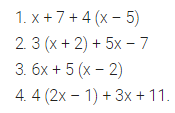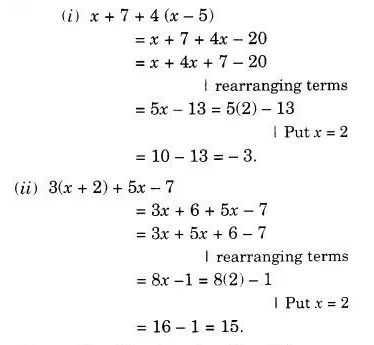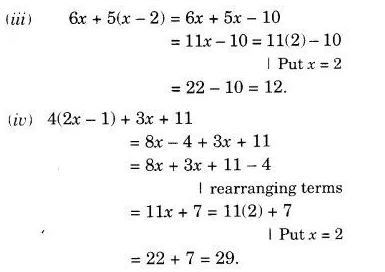# Simplify the expressions and find the value if x is equal to 2. (i) x + 7 + 4 (x – 5)

Simplify the expressions and find the value if x is equal to 2.